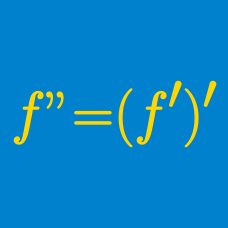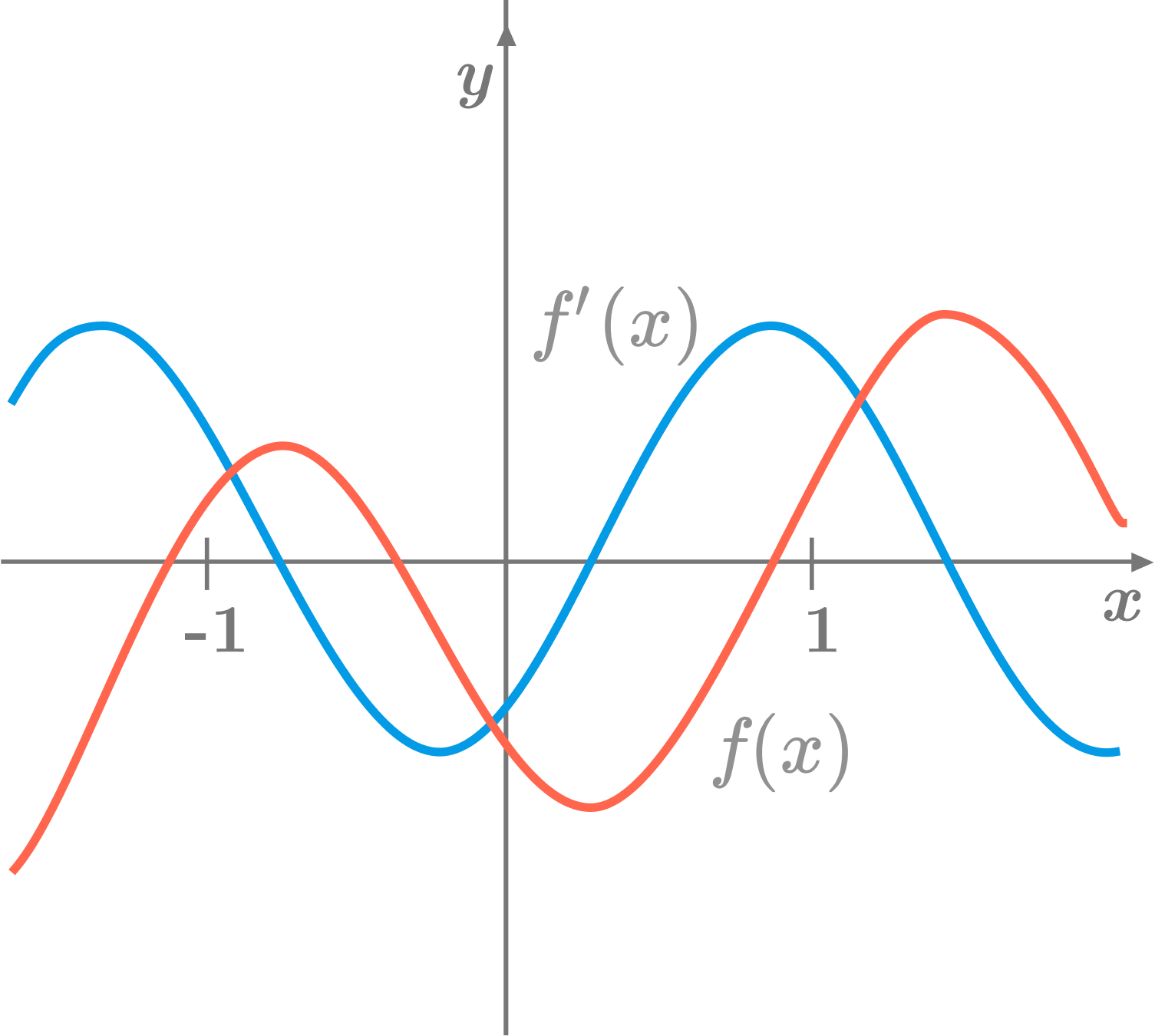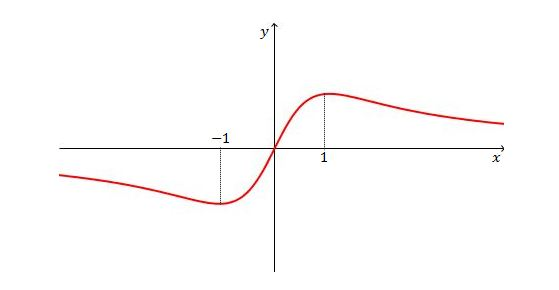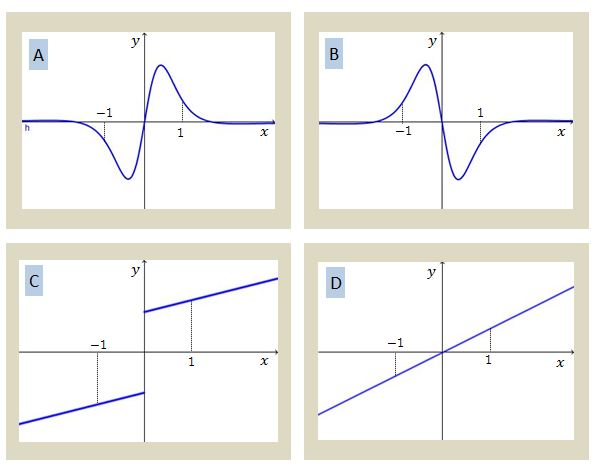Calculus

# Higher-order Derivatives: Level 1 Challenges

What is the fewest number of derivatives one needs to compute so that $f(x) = 568{ x }^{ 499 }$ becomes zero?In the above diagram, the red curve is the graph of a function $f,$ and the blue curve is the graph of its first derivative $f'.$ What is the relationship between $f'(-1)$ and $f''(1) ?$Given the graph of $y=f(x)$ above, which of the following is a possible graph of $y=f''(x)?$Let $P$ be a point moving in the $xy$-plane whose coordinates at time $t$ are given by $x(t)=2e^t\sin t, y(t)=6\cos t.$ What is the minimum value of the magnitude of the acceleration of $P?$

Find $f^{(100)}(0)$. If

$\large{ f(x) = \sin x + x^{100000}}$

Note: $f^n(x)$ denotes the $n^{th}$ derivative of $f(x)$.

×